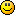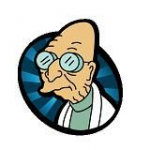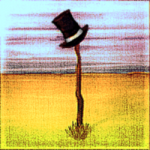BASIC

###Author Topic: Page flipping animation  (Read 5208 times)

####Kitty Hello

• code monkey
• Prof. Inline
••• Posts: 10697
• here on my island the sea says 'hello'##### Page flipping animation
« on: 2010-Apr-06 »
Code: GLBasic [Select]
// --------------------------------- //
// Project: PageFlip
// Start: Tuesday, April 06, 2010
// IDE Version: 7.308

SYSTEMPOINTER TRUE

FOR i=0 TO 999
DRAWRECT RND(500), RND(500), 32, 32, RGB(RND(255), RND(128), RND(255) )
NEXT
GRABSPRITE 0, 0,0, 512, 512
DRAWRECT 0,0,600,600,RGB(255,255,255)
FOR i=0 TO 999
PRINT "Page 2", RND(500), RND(500)
NEXT
GRABSPRITE 1, 0,0, 512, 512

// flip thingy
WHILE TRUE
LOCAL mx%, my%, b1%, b2%
MOUSESTATE mx, my, b1, b2

DRAWRECT 0,0,777,777,RGB(128,128,128)
FlipPageDraw(0, 50,50,480,320, mx, my, FALSE)
SHOWSCREEN
WEND

//! Draws a Flip-Page animation frame
// \param x%,y% - position of left/top page sheet
// \param w%,h% - width/height of the paper sheet -> these coords are used as texture points, too -> texture can be bigger, but starts at 0,0!
// \param px%,py% - point where you "drag" the bottom right corner to
FUNCTION FlipPageDraw%: id%, x, y, w, h, px, py, bLeftToRight%

// draw a frame for where the paper would be
DRAWRECT x,y,w,h, RGB(0xaa, 0xaa, 0xaa)

IF bLeftToRight%
INC x, w
w=-w
ENDIF

IF (w>0 AND px >= x+w) OR (w<0 AND px <=x+w)  // tear the paper to the right
STARTPOLY id, 0
POLYVECTOR x,y, x,y,0xffffff
POLYVECTOR x,y+h,x,y+h,0xffffff
POLYVECTOR x+w,y+h,x+w,y+h,0xffffff
POLYVECTOR x+w,y,x+w,y,0xffffff
ENDPOLY
RETURN
ENDIF

// Find the flip axis
LOCAL fcx, fcy // flip center point. Center between bottom right corner and mouse pos (on bending line)
fcx = (x+w+px)/2
fcy = (y+h+py)/2

// now get the direction of the flip edge (normal to the line px,py -> x+w,y+h)
LOCAL nx, ny
nx = y+h - py
ny = -(x+w - px)

// normalize
LOCAL nl = SQR(nx*nx + ny*ny)
IF nl < 1E-5 THEN RETURN // nothing visible
nx = nx / nl
ny = ny / nl

LOCAL tx, ty // top point (corner of flipped paper)
LOCAL t2x, t2y // 2nd top point (if the bending line hits the top edge, intersection of bottom paper top and bending line)
LOCAL bx, by // bottom point

// if nx=0 we get div/0 -> make a very small value
IF ABS(nx)<=1E-5 THEN nx=1E-4
IF ABS(ny)<=1E-5 THEN ny=1E-4

// Get intersection y or bending line with the bottom paper edge
u = (y+h-fcy)/ny
bx = fcx+u*nx
by = fcy+u*ny

// top point, intersection with right edge
// px + u*nx = x+w
LOCAL u = (x+w-fcx)/nx
ty = fcy + u*ny

LOCAL ty_first = ty

// py+u*ny = y
u = (y-fcy)/ny // intersection of bending line with top edge
t2x = fcx+u*nx
t2y = y

// find the real top point now
// That's a bit tricky now
// the folded edge is perpendicular to the bottom/mouse point line
// _and_ the length equals the distance of the current top point to top/right corner

LOCAL dx, dy // direction of bottom intersection point to mouse point
dx = px-bx
dy = py-by
nl = SQR(dx*dx+dy*dy)
dx=dx/nl
dy=dy/nl

// length of bended page at top edge
u = x+w - t2x
IF w<0 THEN u=-u // flip direction if turning page left to right

tx = t2x+dx * u // move top bending intersection perpendicular to lower teared paper edge
ty = t2y+dy * u

// dont' fold paper too much off the right
// OR  we're far too left with the mouse
IF (w>0 AND tx > x+w) OR (w<0 AND tx < x+w) OR (py<y+h AND ty>y)
tx = x+w
ty = ty_first

t2x=tx
t2y=ty
ENDIF

// Draw the front of that page
STARTPOLY id, 0
POLYVECTOR t2x,t2y,t2x,t2y,0xffffff
POLYVECTOR bx, by, bx ,by ,0xffffff
POLYVECTOR x, y+h, x  ,y+h,0xffffff
POLYVECTOR x, y,   x  ,y  ,0xffffff
POLYVECTOR tx, y,  tx ,y  ,0xffffff
ENDPOLY

// Draw the fine back of the page
// b, p, t - triangle
STARTPOLY -1, 0
POLYVECTOR tx, ty, 0,0,0xffffff
POLYVECTOR t2x,t2y,0,0,0xcccccc
POLYVECTOR bx, by, 0,0,0xcccccc
POLYVECTOR px, py, 0,0,0xffffff
ENDPOLY

ENDFUNCTION

Still some work to do to make it look really well - but it's a start...

 Option to flip from left to right added.
« Last Edit: 2011-Jun-30 by Kitty Hello »

####thomasp

• Mc. Print
•• Posts: 14##### Re: Page flipping animation
« Reply #1 on: 2010-Apr-06 »
Schick!####bigsofty

• Community Developer
• Prof. Inline
••• Posts: 2613##### Re: Page flipping animation
« Reply #2 on: 2010-Apr-06 »
Very cool effect!
Cheers,

Ian.

“It is practically impossible to teach good programming style to students that have had prior exposure to BASIC.  As potential programmers, they are mentally mutilated beyond hope of regeneration.”
(E. W. Dijkstra)

####Hatonastick

• Dr. Type
••• Posts: 474Brilliant!  I love that effect mate.